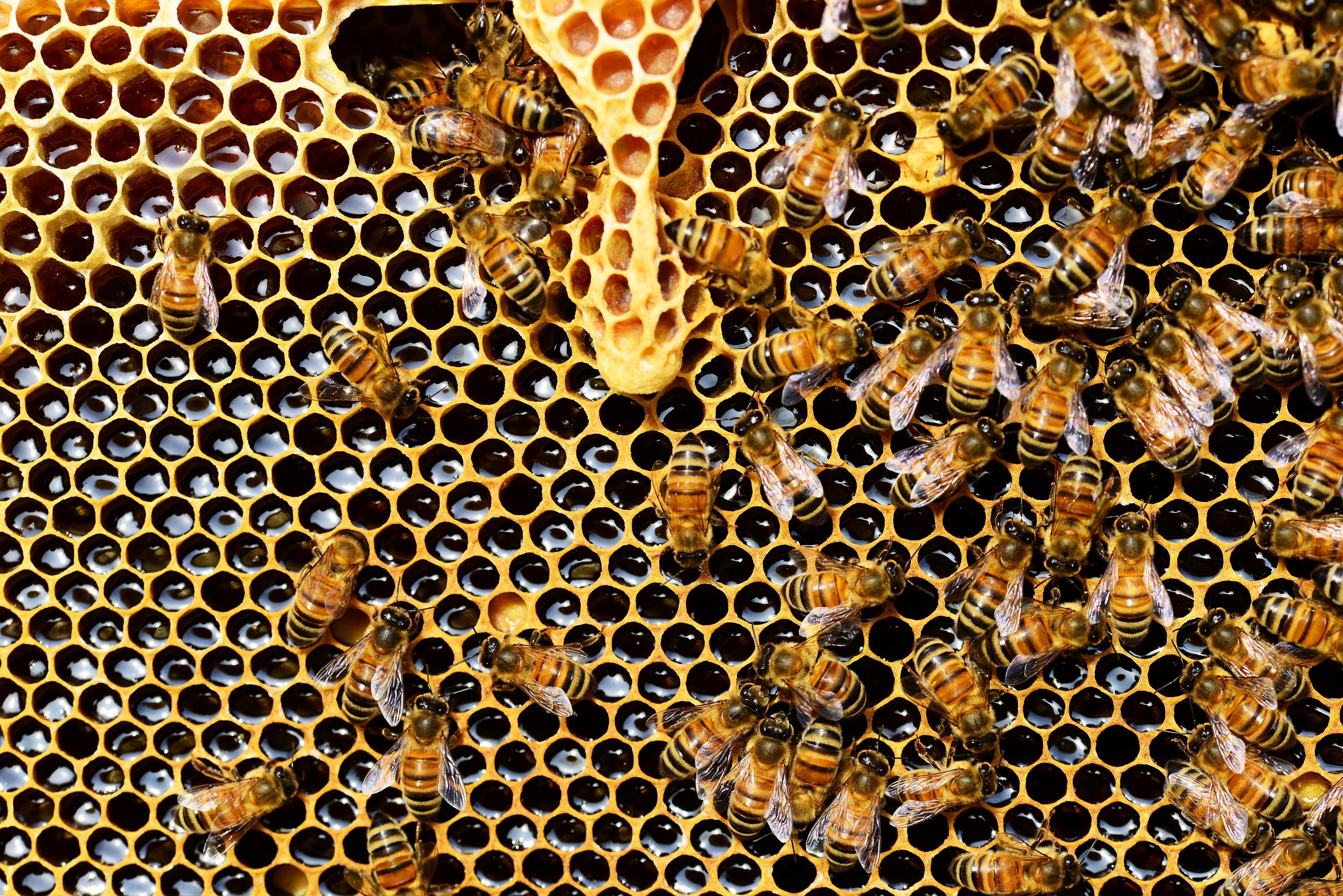# Grid search for parameter tunning

Original article was published by Magdalena Konkiewicz on Artificial Intelligence on MediumGridSearchCV code example

In order to illustrate let’s load the Iris data set. This data set has 150 examples of three different Iris species. The data set has no missing values so there will be no data cleaning needed.

`from sklearn.datasets import load_irisimport pandas as pd%matplotlib inlinedata = load_iris()df = pd.DataFrame(data['data'], columns=data['feature_names'])df['species'] = data['target']df.head()`

Now let’s divide our data set to train and test.

`from sklearn.model_selection import train_test_splitx_train, x_test, y_train, y_test = train_test_split(df.drop('species', axis=1), df.species ,test_size = 0.2, random_state=13)`

Once we have divided the data set we can set up the grid-search with the algorithm of our choice. In our case, we will use it to tune the random forest classifier.

`from sklearn.model_selection import GridSearchCVfrom sklearn.ensemble import RandomForestClassifierrfc = RandomForestClassifier()grid_values = {'n_estimators': [10, 30, 50, 100],               'max_features': ['sqrt', 0.25, 0.5, 0.75, 1.0],               'max_depth' : [4,5,6,7,8],              }grid_search_rfc = GridSearchCV(rfc, param_grid = grid_values, scoring = 'accuracy')grid_search_rfc.fit(x_train, y_train)`

In the code above we first set up the Random Forest Classifier by using a constructor with no parameters. Then we define parameters and the values to try for each parameter in the grid_values variable. ‘grid_values’ variable is then passed to the GridSearchCV together with the random forest object (that we have created before) and the name of the scoring function (in our case ‘accuracy’). Last, by not least we fit it all by calling the fit function on the grid search object.

Now in order to find the best parameters, you can use the best_params_ attribute:

`grid_search_rfc.best_params_`

We are getting the highest accuracy with the trees that are six levels deep, using 75 % of the features for max_features parameter and using 10 estimators.

This has been much easier than trying all parameters by hand.

Now you can use a grid search object to make new predictions using the best parameters.

`grid_search_rfc = grid_clf_acc.predict(x_test)`

And run a classification report on the test set to see how well the model is doing on the new data.

`from sklearn.metrics import classification_reportprint(classification_report(y_test, predictions))`

You can see detailed results for accuracy, recall, precision, and f-score for all of the classes.

Note that we have used accuracy for tuning the model. This may not be the best choice. We can actually use other metrics such as precision, recall, and, f-score. So let’s do that.

`from sklearn.metrics import make_scorer, accuracy_score, precision_score, recall_score, f1_scorescoring = {'accuracy': make_scorer(accuracy_score),           'precision': make_scorer(precision_score, average = 'macro'),           'recall': make_scorer(recall_score, average = 'macro'),           'f1': make_scorer(f1_score, average = 'macro')}grid_search_rfc = GridSearchCV(rfc, param_grid = grid_values, scoring = scoring, refit='f1')grid_search_rfc.fit(x_train, y_train)`

In the code above we set up four scoring metrics: accuracy, precision, recall, and f-score and we store them in the list that is later on passed to grid search as a scoring parameter. We also set the refit parameter to be equal to one of the scoring functions. This is f-score is our case.

`grid_search_rfc.best_params_`
`grid_search_rfc.cv_results_`
`grid_search_rfc.cv_results_['mean_test_recall']`